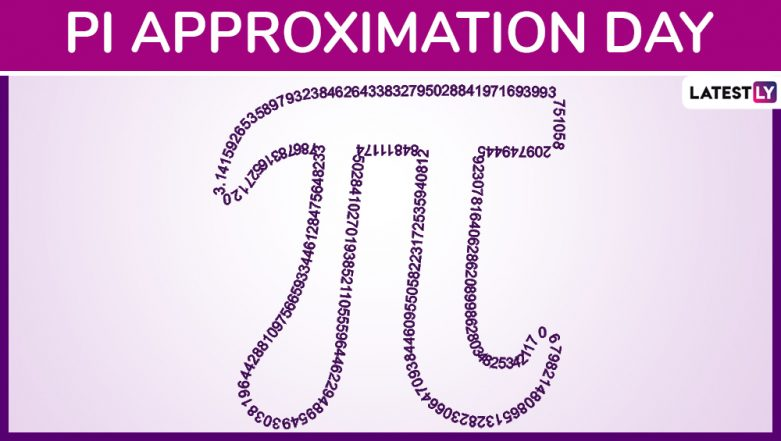Pi Approximation Day (Photo Credits: File Image)

For the nerds and lover of mathematics, it is an important day today, the Pi Approximation Day. The Pi is denoted by the fraction 22/7 thus July 22 is marked as Pi Approximation Day. While Pi Day is marked annually on March 14 denoting the 3.14 calculation. The other approximate value is celebrated with Pi Approximation Day. Pi or π, is an irrational number and a mathematical constant. Pi is defined as the ratio between a circle’s circumference and its diameter. On Pi Approximation Day 2019, we give you some facts about this mathematical constant. Pi Day 2019: Know All About the Day As Math Jokes and Funny Memes Flood Internet.

## Facts About Pi Approximation Day

• There is not one but two days related to pi in a year. Other than Pi day on March 14, there is also the Two Pi Day which is observed on June 28. Sometimes, the entire month of March is observed as Pi Month.
• The first observation regarding Pi was in the year 1988 by Larry Shaw, a physicist at the Exploratorium who revealed it was the day of Pi, March 14.
• While Pi has its mathematical connotation, the celebrations often involve celebrating with Pies the homophone of Pi. So people often bake and eat sweet, round pies.
• Another interesting observation is that when 3:14 is seen in a mirror it reads similar to PIE.
• Pi is very much found in nature if you carefully observe. It is said to be in our cells, the pupils in our eyes, a rainbow and many other things in nature.
• The Pi is indeed never-ending and never-repeating number and it is determined to 12 trillion digits after the decimal point!
• On Pi Day 2019, Google employee Emma Haruka created a new world record of calculating Pi up to 22.4 trillion digits long.

Pi Approximation Day celebrations are similar to Pi Day, since it is only a day that denotes the value of the mathematical constant. While some nerds may participate in remembering the idea of the pi value, others can just look at it as a reason to gorge on sweet pies!